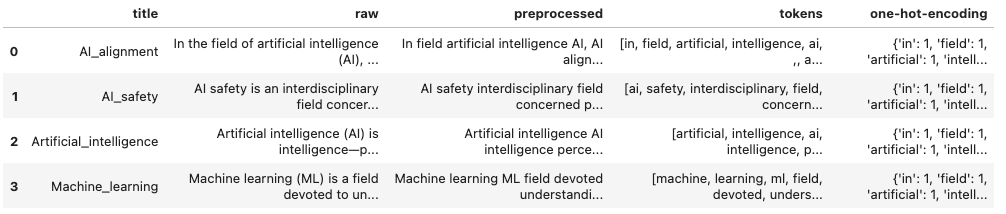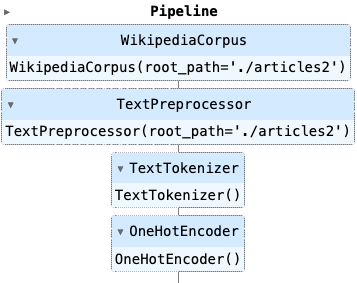# NLP Project: Wikipedia Article Crawler & Classification - Corpus Transformation Pipeline

Posted in Nlp, Python, Wikipedia_crawler

My NLP project downloads, processes, and applies machine learning algorithms on Wikipedia articles. In my last article, the projects outline was shown, and its foundation established. First, a Wikipedia crawler object that searches articles by their name, extracts title, categories, content, and related pages, and stores the article as plaintext files. Second, a corpus object that processes the complete set of articles, allows convenient access to individual files, and provides global data like the number of individual tokens.

In this article, I continue show how to create a NLP project to classify different Wikipedia articles from its machine learning domain. You will learn how to create a custom SciKit Learn pipeline that uses NLTK for tokenization, stemming and vectorizing, and then apply a Bayesian model to apply classifications. All code can also be seen in a Jupyter Notebook.

The technical context of this article is `Python v3.11` and several additional libraries, most important `pandas v2.0.1`, `scikit-learn v1.2.2`, and `nltk v3.8.1`. All examples should work with newer versions too.

## Requirements and Used Python Libraries

Be sure to read and run the requirements of the previous article in order to have a Jupyter Notebook to run all code examples.

Each of these steps will become part of a pipeline objects, a sequential process that reads, pre-processes, vectorizes and clusters text. We will use the following Python libraries and objects in this project:

• Pandas
• `DataFrame` objects to store the text, tokens, and vectors
• SciKitLearn
• `Pipeline` object to implement the chain of processing steps
• `BaseEstimator` and `TransformerMixin` to build custom classes that represent Pipeline steps
• NLTK
• `PlaintextCorpusReader` for a traversable object that gives access to documents, provides tokenization methods, and computes statistics about all files
• `sent_tokenizer` and `word_tokenizer` for generating tokens
• The `stopwords` list for token reduction

## SciKit Learn Pipeline

To facilitate getting consistent results and easy customization, SciKit Learn provides the Pipeline object. This object is a chain of transformers, objects that implement a `fit` and `transform` method, and a final estimator that implements the `fit` method. Executing a pipeline object means that each transformer is called to modify the data, and then the final estimator, which is a machine learning algorithm, is applied to this data. Pipeline objects expose their parameter, so that hyperparameters can be changed or even whole pipeline steps can be skipped.

We will use this concept to build a pipeline that starts to create a corpus object, then preprocesses the text, then provide vectorization and finally either a clustering or classification algorithm. To keep the scope of this article focused, I will only explain the transformer steps, and approach clustering and classification in the next articles.

## Pipeline Preparation

Lets begin with the big-picture. The final pipeline object will be implemented as follows:

``````pipeline = Pipeline([
('corpus', WikipediaCorpus()),
('preprocess', TextPreprocessor()),
('tokenizer', Tokenizer()),
('encoder', OneHotEncoder())
])
``````

This pipeline then starts with an empty Pandas DataFrame object to which data is added subsequently, that is we achieve a DataFrame object that looks like this:For each of these steps, we will use a custom class the inherits methods from the recommended ScitKit Learn base classes.

``````from sklearn.base import BaseEstimator, TransformerMixin
from nltk.tokenize import sent_tokenize, word_tokenize

class SciKitTransformer(BaseEstimator, TransformerMixin):
def fit(self, X=None, y=None):
return self

def transform(self, X=None):
return self
``````

Let’s start the implementation.

## Pipeline Step 1: Creating the Corpus

The first step is to reuse the Wikipedia corpus object that was explained in the previous article, and wrap it inside out base class, and provide the two DataFrame columns `title` and `raw`. In the title column, we store the filename except the `.txt` extension. In the `raw` column, we store the complete content of the file.

This transformation uses list comprehensions and the built-in methods of the NLTK corpus reader object.

``````class WikipediaCorpus(PlaintextCorpusReader):
def __init__(self, root_path):

class WikipediaCorpus(SciKitTransformer):
def __init__(self, root_path=''):
self.root_path = root_path

def transform(self, X=None):
X = pd.DataFrame().from_dict({
'title': [filename.replace('.txt', '') for filename in self.corpus.fileids()],
'raw': [self.corpus.raw(doc) for doc in corpus.fileids()]
})
return X
``````

## Pipeline Step 2: Text Preprocessing

In NLP applications, the raw text is typically checked for symbols that are not required, or stop words that can be removed, or even applying stemming and lemmatization.

For the Wikipedia articles, I decided to separate the text into sentences and token, than token transformations, and finally put it all together again. The transformations are these:

• remove all stopwords
• remove all non-ascii-alphabet, non-numbers token
• keep only `,`. `;`, and `.` for sequence delimitation
• Remove all occurrences of multiple whitespace with a single whitespace

Here is the complete implementation of the `TextPreprocessor`. The DataFrame object is extended with the new column `preprocessed` by using Pandas `apply` method.

``````class TextPreprocessor(SciKitTransformer):
def __init__(self, root_path=''):
self.root_path = root_path
self.tokenizer = word_tokenize

def preprocess(self, text):
preprocessed = ''
for sent in sent_tokenize(text):
if not len(sent) <= 3:
text = ' '.join([word for word in word_tokenize(sent) if not word in stopwords.words("english")])
text = re.sub('[^A-Za-z0-9,;\.]+', ' ', text)
text = re.sub(r'\s+', ' ', text)
# preserve text tokens
text = re.sub(r'\s\.', '.', text)
text = re.sub(r'\s,', ',', text)
text = re.sub(r'\s;', ';', text)
# remove all non character, non number chars
preprocessed += ' '+ text.strip()
return preprocessed

def transform(self, X):
X['preprocessed'] = X['raw'].apply(lambda text: self.preprocess(text))
return X
``````

## Pipeline Step 3. Tokenization

The preprocessed text is now tokenized again, using the same NLT `word_tokenizer` as before, but it can be swapped with a different tokenizer implementation.

As before, the DataFrame is extended with a new column, `tokens`, by using `apply` on the `preprocessed` column.

``````class TextTokenizer(SciKitTransformer):
def preprocess(self, text):
return [token.lower() for token in word_tokenize(text)]

def transform(self, X):
X['tokens'] = X['preprocessed'].apply(lambda text: self.preprocess(text))
return X

``````

## Pipeline Step 4: Encoder

Encoding a tokenized text is the precursor to vectorization. To keep this article focused, I will provide a rather simple encoding method, which computes the complete vocabulary of all texts and one-hot encodes all words that appear in a specific article. The base for the vocabulary is opiniated: I use the list of refined tokens as input, but one could also use the `vocab` method from the NLTK `CorpusReader` object.

``````class OneHotEncoder(SciKitTransformer):
def encode(self, token_series, tokens):
one_hot = {}
for _, token_list in token_series.items():
for token in token_list:
one_hot[token] = 0

for token in tokens:
one_hot[token] = 1

return one_hot

def transform(self, X):
token_list = X['tokens']
X['one-hot-encoding'] = X['tokens'].apply(lambda tokens: self.encode(token_list, tokens))
return X
``````

This encoding is very costly because the complete vocabulary is built from scratch for each run - something that can be improved in future versions.

## Complete Source Code

Here is the complete example:

``````import numpy as np
import pandas as pd

from nltk.tokenize import sent_tokenize, word_tokenize
from nltk.corpus import stopwords

from sklearn.base import TransformerMixin
from sklearn.pipeline import Pipeline

from nltk.tokenize.stanford import StanfordTokenizer

def __init__(self, root_path):

class SciKitTransformer(BaseEstimator, TransformerMixin):
def fit(self, X=None, y=None):
return self

def transform(self, X=None):
return self

class WikipediaCorpus(SciKitTransformer):
def __init__(self, root_path=''):
self.root_path = root_path
self.wiki_corpus = WikipediaPlaintextCorpus(self.root_path)

def transform(self, X=None):
X = pd.DataFrame().from_dict({
'title': [filename.replace('.txt', '') for filename in self.wiki_corpus.fileids()],
'raw': [self.wiki_corpus.raw(doc) for doc in corpus.fileids()]
})
return X

class TextPreprocessor(SciKitTransformer):
def __init__(self, root_path=''):
self.root_path = root_path
self.corpus = WikipediaPlaintextCorpus(self.root_path)

def preprocess(self, text):
preprocessed = ''
for sent in sent_tokenize(text):
text = ' '.join([word for word in word_tokenize(sent) if not word in stopwords.words("english")])
text = re.sub('[^A-Za-z0-9,;\.]+', ' ', text)
text = re.sub(r'\s+', ' ', text)
# preserve text tokens
text = re.sub(r'\s\.', '.', text)
text = re.sub(r'\s,', ',', text)
text = re.sub(r'\s;', ';', text)
# remove all non character, non number chars
preprocessed += ' '+ text.strip()
return preprocessed

def transform(self, X):
X['preprocessed'] = X['raw'].apply(lambda text: self.preprocess(text))
return X

class TextTokenizer(SciKitTransformer):
def preprocess(self, text):
return [token.lower() for token in word_tokenize(text)]

def transform(self, X):
X['tokens'] = X['preprocessed'].apply(lambda text: self.preprocess(text))
return X

class OneHotEncoder(SciKitTransformer):
def encode(self, token_series, tokens):
one_hot = {}
for _, token_list in token_series.items():
for token in token_list:
one_hot[token] = 0

for token in tokens:
one_hot[token] = 1

return one_hot

def transform(self, X):
token_list = X['tokens']
X['one-hot-encoding'] = X['tokens'].apply(lambda tokens: self.encode(token_list, tokens))
return X

corpus = WikipediaPlaintextCorpus('articles')

pipeline = Pipeline([
('corpus', WikipediaCorpus(root_path='./articles')),
('preprocess', TextPreprocessor(root_path='./articles')),
('tokenizer', TextTokenizer()),
('encoder', OneHotEncoder())
])
``````

The pipeline object is rendered in the Jupyter Notebook like this:## Conclusion

The SciKit Learn `Pipeline` object provides a convenient way to stack multiple transformations and a machine learning model together. All relevant hyperparameters can be exposed and configured to obtain repeatable results. In this article, you learned how to create a text processing pipeline for Wikipedia articles with four steps: a) `WikipediaCorpus` for accessing plaintext files and global statistics like word occurrences, b) `TextPreprocessor` for removing symbols and stop words from the texts, c) `TextTokenizer` to creating tokens from the preprocessed text, and d) `OneHotEncoder` to provide a simple statistic which words from the total corpus vocabulary appear in a specific article. The next article continues how to transform the tokens and encodings to numerical vector representations.